» Experiment » Calculator/Converters » Radio » Newsletter » Associations and Societies » Component Manufacturers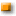Direct CurrentAlternating CurrentDigital ElectronicsReferencesExperimentComputer ArchitecturesElectronics Symentics A B C D E F G H I J K L M N O P Q R S T U V W X Y Z

Electronic formulas

Ohm's Law Formulas for D-C Circuits: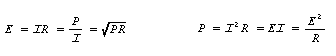Ohm's Law Formulas for A-C Circuits:

In these formulas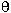is the angle of lead or lag between current and voltage and cos= P/EI = power factor.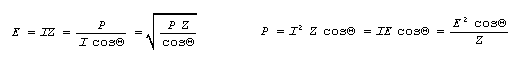Resistors in Series: Rtotal = R1 + R2 + R3 + . . . .

Two Resistors in Parallel: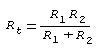Resistors in Parallel, General Formula: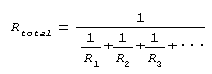Sinusoidal Voltages and Currents:

 Effective value [Also known as Root-Mean Square (RMS) value] = 0.707 x peak value Half Cycle Average value = 0.637 x peak value Peak value = 1.414 x effective value Effective value = 1.11 x average value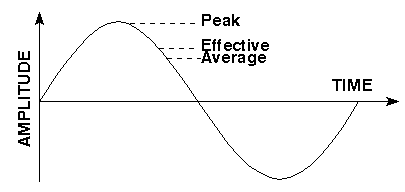Resonant Frequency Formulas: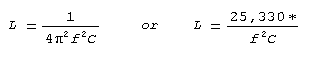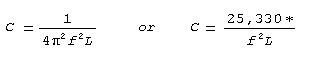*Where in the second formula f is in kHz and L and C are in microunits.

Conductance: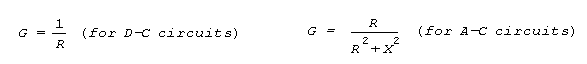Reactance Formulas: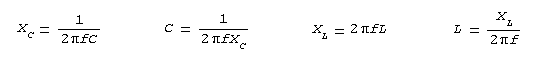Impedance Formulas: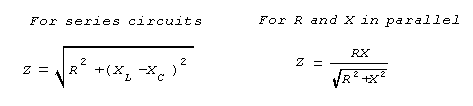Q or Figure of Merit: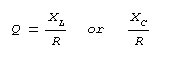Voltage/Current Phase Rule of Thumb:

Remember "ELI the ICE man"

ELI: Voltage (E) comes before (leads) current (I) in an inductor (L)

ICE: Current (I) comes before (leads) Voltage (E) in a capacitor (C)